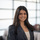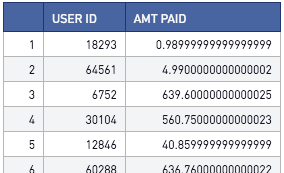# Calculating Trimmed Means (SQL and Python Variations)

Data can oftentimes have extreme outliers, which can heavily skew certain metrics, such as the mean. One way to get around this is to using a trimmed mean. Using a trimmed mean, users will remove the top and bottom x percent of their data and take the average of the result.

We see trimmed ranges as a standard methodology that college programs use to communicate the standardized test scores of their admitted students (ex: 25th to 75th percentile of SAT scores). This is the same thought process that drives some data professionals to use trimmed means.

For the examples below, let's assume we have the following data: user_ids, and how much money they spent on a fictional gaming app.

Figure 1Note: There are a few nuances to keep in mind when developing a protocol for trimmed means. Primarily, we need to assess how to handle multiple occurrences of a value when it is either the lower or upper bound of results to be trimmed.

Case 1: Exclude upper and lower bounds of the trimmed dataset

You can perform this calculation in Redshift SQL or Python

Redshift SQL (assume the table in Figure 1 is stored in t1)

```with t2 as (select
case
when amt_paid <= PERCENTILE_DISC(0.1) WITHIN group(order by amt_paid) over()
then null
when amt_paid >= PERCENTILE_DISC(1-0.1) WITHIN group(order by amt_paid) over()
then null
else amt_paid end as trimmed_vals
from
t1)
select avg(trimmed_vals) from t2 ```

Python 3.6 (assume the table in Figure 1 is stored in a dataframe df)

```# SQL output is imported as a pandas dataframe variable called "df"

# Source:  https://stackoverflow.com/questions/19441730/trimmed-mean-with-percentage-limit-in-python
import pandas as pd
import matplotlib.pyplot as plt
from scipy.stats import tmean, scoreatpercentile
import numpy as np

def trimmean(arr, percent):
lower_limit = scoreatpercentile(arr, percent)
upper_limit = scoreatpercentile(arr, 100-percent)
return tmean(arr, limits=(lower_limit, upper_limit), inclusive=(False, False))

my_result = trimmean(df["amt_paid"].values,10)```

Case 2: Disregard duplicate values of the upper/lower bounds

In other words, ensure that exactly x% of the lowest and x% of the highest values are removed from the dataset. If there are multiple records where the value is equal to the upper limit, discard enough copies such that the resulting sample size is 2*x% smaller than the initial sample size.

SQL

There is no known effective SQL equivalent for this behavior

Python 3.6

```# SQL output is imported as a pandas dataframe variable called "df"
import pandas as pd
from scipy.stats import trim_mean
import numpy as np

my_result = trim_mean(df["amt_paid"].values, 0.1)```

Case 3: Include upper and lower bounds of the trimmed dataset

Redshift SQL (assume the table in Figure 1 is stored in t1)

```with t2 as (select
case
when amt_paid < PERCENTILE_DISC(0.1) WITHIN group(order by amt_paid) over()
then null
when amt_paid > PERCENTILE_DISC(1-0.1) WITHIN group(order by amt_paid) over()
then null
else amt_paid end as trimmed_vals
from
t1)
select avg(trimmed_vals) from t2 ```

Python 3.6 (assume the table in Figure 1 is stored in a dataframe df)

```# SQL output is imported as a pandas dataframe variable called "df"

# Source:  https://stackoverflow.com/questions/19441730/trimmed-mean-with-percentage-limit-in-python
import pandas as pd
import matplotlib.pyplot as plt
from scipy.stats import tmean, scoreatpercentile
import numpy as np

def trimmean(arr, percent):
lower_limit = scoreatpercentile(arr, percent)
upper_limit = scoreatpercentile(arr, 100-percent)
return tmean(arr, limits=(lower_limit, upper_limit), inclusive=(True, True))

my_result = trimmean(df["amt_paid"].values,10)```